In: Chemistry

# 11 mL of 0.0100 M HCl are added to 25.0 mL of a buffer solution that...

11 mL of 0.0100 M HCl are added to 25.0 mL of a buffer solution that is 0.010 M HC2H3O2 and 0.08 M C2H3O2-.  What is the pH of the resulting solution?

## Solutions

##### Expert Solution

Number of mmol of HCl added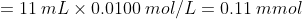Number of mmol of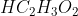added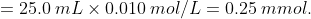Number of mmol of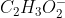added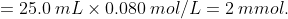0.11 mmol of HCl will react with 0.11 mmol ofto form 0.11 mmol of.

Total number of mmol ofTotal number of mmol ofTotal volume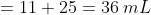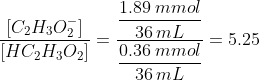For acetic acid, pKa is 4.74.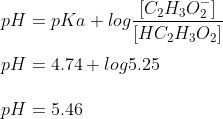## Related Solutions

##### If 100.mL of 0.035 M HCl solution is added to 220.mL of a buffer solution which...
If 100.mL of 0.035 M HCl solution is added to 220.mL of a buffer solution which is 0.20M in NH3 and 0.18M in NH4Cl, what will be the pH of the new solution? The Kb of NH3 is 1.8x10^-5. Please explain how the answer was obtained. Thank you!
##### Suppose 50.00 mL of 0.300 M HCl is added to an acetate buffer prepared by dissolving...
Suppose 50.00 mL of 0.300 M HCl is added to an acetate buffer prepared by dissolving 0.100 mol of acetic acid and 0.110 mol of sodium acetate in 0.100 L of solution. What are the initial and final pH values? What would be the pH if the same amount of HCl solution were added to 170 mL of pure water?
##### A student added 50.0 mL of an NaOH solution to 100.0 mL of 0.300 M HCl....
A student added 50.0 mL of an NaOH solution to 100.0 mL of 0.300 M HCl. The solution was then treated with an excess of aqueous chromium(III) nitrate, resulting in formation of 2.36 g of precipitate. Determine the concentration of the solution.
##### if 0.050 mol of hcl is added to 1.0 l of buffer solution containing 0.125 M...
if 0.050 mol of hcl is added to 1.0 l of buffer solution containing 0.125 M potassium acetate and 0.10 M acetic acid what is the change in PH of the solution
##### Ascorbic asic (0.0100 M) was added to 10.0 mL of 0.0200 M Fe3+ at pH =...
Ascorbic asic (0.0100 M) was added to 10.0 mL of 0.0200 M Fe3+ at pH = 0.30, and the potential was monitored with Pt and saturated Ag/AgCl electrodes. Dehydroascorbic acid + 2H+ + 2e- --> ascorbic acid + H2O (Eo = 0.390 V) Using Eo = 0.767 V for the Fe3+/Fe2+ couple, calculate the cell voltage when 5.0, 10.0, and 15.0 mL of ascorbic acid have been added. Correct answers are: 0.570 V, 0.307 V, and 0.184 V but not...
##### 50 mL of 2.0 M NaOH is added to a 1.0L buffer solution that is 0.20M...
50 mL of 2.0 M NaOH is added to a 1.0L buffer solution that is 0.20M in HF and 0.20M in KF. The ka for HF is 3.5 x 10^-4. 1. Write the reaction or the buffer only including states and excluding spectator ions. 2. Write the neutralization reaction that occurs after the addition of LiOH including states and excluding spectator ions. 3. Calculate the amount of moles remaining and the final concentration for each component of the solution after...
##### Calculate the pH of the resulting solution if 27.0 mL of 0.270 M HCl(aq) is added...
Calculate the pH of the resulting solution if 27.0 mL of 0.270 M HCl(aq) is added to (a) 37.0 mL of 0.270 M NaOH(aq). (b) 17.0 mL of 0.370 M NaOH(aq).
##### To a 250.0 mL volumetric flask are added 1.00 mL volumes of three solutions: 0.0100 M...
To a 250.0 mL volumetric flask are added 1.00 mL volumes of three solutions: 0.0100 M AgNO3, 0.150M NaBr, and 0.100 M NaCN. The mixture is diluted with deionized water to the mark and shaken vigorously. The Ksp of AgBr is 5.40×10–13 and the Kf of Ag(CN)2– is 1.00×1021. What mass of AgBr would precipitate from this mixture? Do not simply write "0" as some precipitate will form.
##### 25.0 mL of a 0.100 M NH3 is titrated with a strong acid. 0.100 M HCl....
25.0 mL of a 0.100 M NH3 is titrated with a strong acid. 0.100 M HCl. Calculate the pH of the NH3 solution at the following points during the titration: (Kb= 1.8 x 10^-5) A. Prior to the addition of any HCl. B: After the addition of 10.5 mL of a 0.100 M HCl. C: At the equivilance point. D: After the addition of 3 mL of 0.100 M HCl. Show your work.
##### What is the pH of the solution of 90 mL of 1.1 HCl is added to...
What is the pH of the solution of 90 mL of 1.1 HCl is added to a solution of 250 mL of 1.0 M HCN and 1.0 NaCN? (HCN Ka = 6.2 x10-10)